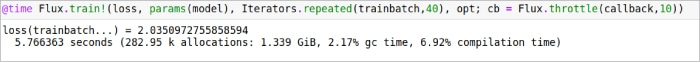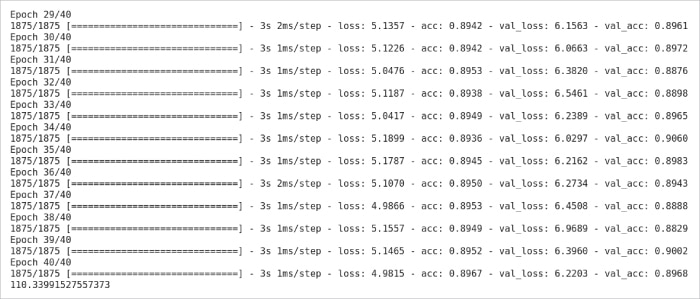HomeAudienceDevelopersJulia and Python: Which Language Is Quicker?

# Julia and Python: Which Language Is Quicker?

Julia is a dynamic programming language with a high level of abstraction. While it is a general-purpose language that may be used to develop any program, it has several characteristics that are ideally suited to numerical analysis and computational research. Python was designed in the early 1990s as a simple object-oriented programming language, but has evolved significantly since then. This article takes a deeper look at the performance of both for neural networks and machine learning.

Julia’s architecture features parametric polymorphism in a dynamic programming language, as well as a multiple dispatch programming paradigm as its primary programming model. It allows concurrent, parallel, and distributed computing with or without message passing interface (MPI) or the built-in ‘OpenMP-style’ threads, as well as the direct invocation of C and FORTRAN libraries without intermediate code. Julia employs a just-in-time (JIT) compiler, which the Julia community refers to as ‘just-ahead-of-time’ (JAOT) since it compiles all code to machine code by default before running it.

Unlike Python, Julia was designed specifically for use in statistics and machine learning.
Julia can fly through linear algebra, whereas Python can plod through it. This is because Python was never designed to accommodate all of the matrices and equations that machine learning requires. Python isn’t bad by any means, especially with NumPy, but Julia is a lot better tailored to this kind of mathematics in terms of a no-package experience. Julia’s operand system is a lot more like R’s than Python’s, which is a significant plus. The majority of linear algebra can be completed in less time and with less effort.

As we know, in recent years Python has dominated the areas of machine learning and data science. Because of the variety of third-party libraries that we can use in Python, it helps to develop machine learning code easily. While there are so many advantages of Python, there is one major drawback — it’s an interpreted language, which makes it slow. This is the age of data, and the more data there is the more time it takes to work on it. That’s where Julia comes into the picture.

Most of the research work on Julia so far has been on topics like high power computing or the scientific calculation capabilities of Julia. But here we will talk about how Julia is not only capable of working on complex scientific calculations efficiently but also on commercial-based problems, and can tackle Python in machine learning and neural networks.

## Objective and experimentation

Julia is as simple as Python but is a compiled language like C. So let’s test how fast Julia is in comparison with Python. For that, we will first test these languages on some simple programs and then move to the main focus of our experiment, which is to test them for machine learning and deep learning.

Julia and Python provide many libraries and open source benchmarking tools. For benchmarking and calculating time in Julia, we used CPUTime and time libraries. Similarly, for Python, we used the time module.

## Matrix multiplication

We tried out simple arithmetic operations first, but since these will not generate much difference in time we decided to check out the timing in matrix multiplication. We started with creating two (10 * 10) matrices of random float numbers and performed dot products in these. As we know, Python has a NumPy library, which is famous for working with matrices and vectors. Similarly, Julia has a LinearAlgebra library that works well with matrices and vectors. So we compared the matrix multiplication with and without using their respective libraries. The source code for all the programs used in this article is available in our GitHub repository (https://github.com/mr-nerdster/Julia_Research.gitsee). The 10×10 matrix multiplication program written in Julia is given below:

```@time LinearAlgebra.mul!(c,x,y)

function MM()
x = rand(Float64,(10,10))
y = rand(Float64,(10,10))
c = zeros(10,10)

for i in range(1,10)
for j in range(1,10)
for k in range(1,10)
c[i,j] += x[i,k]*y[k,j]
end
end
end
end
@time MM

0.000001 seconds
MM (generic function with 1 method)```

Julia takes 0.000017 seconds using the library and 0.000001 seconds using loops.

The same matrix multiplication program was written in Python, as shown below. From the results it can be seen that with the library the program takes less time compared to without the library.

```import numpy as np
import time as t
x = np.random.rand(10,10)
y = np.random.rand(10,10)
start = t.time()
z = np.dot(x, y)
print(“Time = “,t.time()-start)
Time = 0.001316070556640625

import random
import time as t
l = 0
h= 10
cols = 10
rows= 10

choices = list (map(float, range(l,h)))
x = [random.choices (choices , k=cols) for _ in range(rows)]
y = [random.choices (choices , k=cols) for _ in range(rows)]

result = [(*cols) for i in range (rows)]

start = t.time()

for i in range(len(x)):
for j in range(len(y)):
for k in range(len(result)):
result[i][j] += x[i][k] * y[k][j]

print(result)
print(“Time = “, t.time()-start)

Time = 0.0015912055969238281```

Python takes 0.0013 seconds using the library and 0.0015 seconds using loops.

## Linear search

The next experiment that we performed was a linear search on one hundred thousand randomly generated numbers. We used two methods here — one by using a for loop and the other by using an operator. We performed 1000 searches with integers ranging from 1 to 1000, and as you can see in the output below we also printed out how many integers we find in the data set. The output of time by using loops and by using the IN operator is given below. Here we measured time by taking the median CPU time of 3 runs.

The program was written for Julia and the results are shown below.

```import numpy as np
import time as t
x = np.random.rand(10,10)
y = np.random.rand(10,10)
start = t.time()
z = np.dot(x, y)
print(“Time = “,t.time()-start)
Time = 0.001316070556640625

import random
import time as t
l = 0
h= 10
cols = 10
rows= 10

choices = list (map(float, range(l,h)))
x = [random.choices (choices , k=cols) for _ in range(rows)]
y = [random.choices (choices , k=cols) for _ in range(rows)]

result = [(*cols) for i in range (rows)]

start = t.time()

for i in range(len(x)):
for j in range(len(y)):
for k in range(len(result)):
result[i][j] += x[i][k] * y[k][j]

print(result)
print(“Time = “, t.time()-start)

Time = 0.0015912055969238281```

The same program was written for Python and the results are:

```FOR_SEARCH:
Elapsed CPU time: 16.420260511 seconds
matches: 550
Elapsed CPU time: 16.140975079 seconds
matches: 550
Elapsed CPU time: 16.49639576 seconds
matches: 550

IN:
Elapsed CPU time: 6.446583343 seconds
matches: 550
Elapsed CPU time: 6.216615487 seconds
matches: 550
Elapsed CPU time: 6.296716556 seconds
matches: 550```

From the above results, it is evident that there are no time differences between using a loop and an operator in Julia. However, the loop takes almost three times more execution time than the IN operator in Python. The interesting point here is that, in both cases, Julia has a much faster execution time than Python.

## Linear regression

The next experiments were performed in machine learning algorithms. We first worked on one of the most common and simple machine learning algorithms, i.e., linear regression with a simple data set. We used a ‘Head Brain’ data set that contains 237 data entries and has two columns [HeadSize, BrainWeight]. In this, we had to calculate the brain weight by using the head size. So we implemented linear regression from scratch, without using any library on this data set in both Python and Julia.

Julia:

```GC.gc()
@CPUtime begin
linear_reg()
end
elapsed CPU time: 0.000718 seconds```

Python:

```gc.collect()
start = process_time()
linear_reg()
end = process_time()

print(end-start)
elapsed time: 0.007180344000000005```

The time taken by both Julia and Python is given above.

## Logistic regression

Next, we carried out an experiment on the most common type of machine learning algorithm, i.e., logistic regression, by using libraries in both languages. For Python, we used its most commonly used library sklearn while in Julia we used the GLM library. The data set that we used for this is the information about a bank’s clients, which contains 10,000 data entries. The target variable is a binary variable that indicates whether the consumer left the bank (closed his or her account) or remained a customer.

The time taken by Julia for logistic regression is given below.

```@time log_rec()
0.027746 seconds (3.32 k allocations: 10.947 MiB)```

The time taken by Python for logistic regression is also given below.

```gc.collect()
start = process_time()
LogReg()
end = process_time()
print(end-start)

Accuracy : 0.8068
0.34901400000000005```

## Neural networks

After testing both languages on various programs and data sets, we tested them on neural networks and used the MNIST data set. This data set contains gray-scale images of hand-drawn digits, from zero through nine. Each image is 28×28 pixels. Each pixel value indicates the lightness or darkness of that pixel, and this value is an integer between 0 and 255, both inclusive. The data also contains a label column which represents the digit that was drawn in the respected image.

Figure 1 shows a few examples of the MNIST data set.

We created a simple neural network to test the time taken by both languages. The structure of our neural network is like this:

`Input ---> Hidden layer ---> Output`

It contains an input layer, hidden layer, and output layer. To avoid complexities, we did not use any preprocessing on our data set, and worked on it as it is. We trained this model for 40 iterations and checked the time difference between Julia and Python in it.Figure 2: Julia takes 5.76 seconds in a neural network

For Julia, the Flux library was used to implement the neural network and for Python, the Keras library was used. Figure 2 shows the time taken by Julia in a neural network.
Figure 3 shows the time taken by Python and a few iterations of the model in the neural network.Figure 3: Python takes 110.3 seconds in a neural network

The results show that there are huge time differences between Julia and Python when it comes to a neural network.

Table 1 summarises all the results of our experiments, and gives the time difference (in percentage) between Julia and Python.

 Experiment Julia (seconds) Python(seconds) Time difference (%) Matrix multiplication (without library) 0.000001 0.0015 99.9 Matrix multiplication (with library) 0.000017 0.0013 98.69 Linear search (using loop ) 0.42 16.4 97.43 Linear search (using IN operator) 0.43 6.2 93.06 Linear regression 0.000718 0.00718 90 Logistic regression 0.025 0.34901 92.83 Neural networks 5.76 110.3 94.77

All the experiments we carried out indicated that as the complexity of the program as well as the size of the data set increases, the execution time difference between Julia and Python increases. From the results, we can conclude that Julia is the better programming language for machine learning and neural networks.

Previous articleWhere Is DevOps Headed?B Thangaraju
The author is associated with the Open source technology Lab, International Institute of Information Technology, Bengaluru. He can be reached at b.thangaraju@iiitb.ac.in